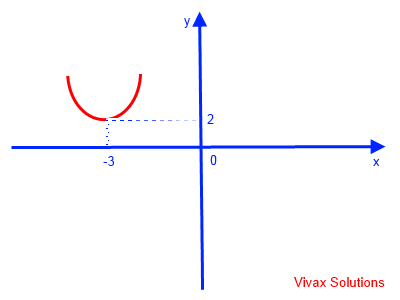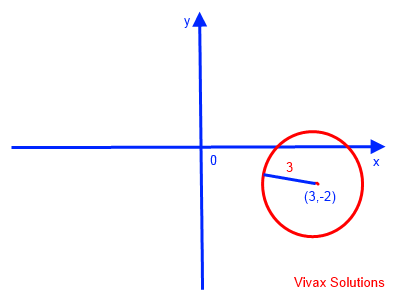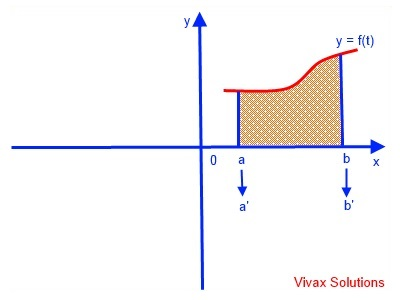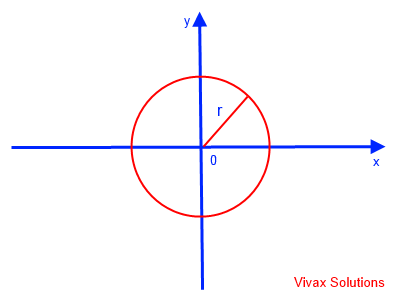### Parametric Equations

When the coordinates of curve are expressed in terms of a third independent variable - parameter - parametric equations are produced.

E.g.

y = 2t; x = t2 - 3

Here, 't' is the parameter.

E.g.1

y = t2 + 2; x = (t-3);
Now, we have a choice - finding the values of x and y manually by substituting the values of t or use algebra to find the Cartesian equation. Let's go for the latter.
t = (x+3) => y =(x + 3)2 + 2
This can easily be sketched: basic quadratic curve translated -3 in the x-axis and 2 in the y axis.E.g.2

y = t; x = (t - 3)2;
t = √x+3 => y = √x + 3
This can easily be sketched as follows:E.g.3

y = sec t; x = cos t
xy = cos t . 1/cos t
xy = 1
y = 1/x This is reciprocal curve.
This can easily be sketched as follows:E.g.4

y = 3 sin t; x = 3 cos t
cos t = x/3; sin t = y/3;
x2 / 9 + y2 / 9 = sin2 t + cos2 t = 1
x2 / 9 + y2 / 9 = 1
x2 + y2 = 9
x2 + y2 = 32
This is a circle with radius 3.
This can easily be sketched as follows:E.g.5

y = -2 + 3 sin t; x = 3 + 3 cos t
cos t = (x-3)/3; sin t = (y+2)/3;
(x-3)2 / 9 + (y+2)2 / 9 = sin2 t + cos2 t = 1
(x-3)2 / 9 + (y+2)2 / 9 = 1
(x-3)sup>2 + (y+2)2 = 9
(x-3)2 + (y+2)2 = 32
This is a circle with the centre at (3,-2) and radius 3.
This can easily be sketched as follows:E.g.6

y = 3 sin t; x = 4 cos t
cos t = x/4; sin t = y)/3;
x2/16 + y2 / 9 = sin2 t + cos2 t = 1
x2 / 16 + y+2 / 9 = 1
if x = 0 => y = +3 or -3
if y = 0 => x = +4 or -4
It is an ellipse. This can easily be sketched as follows:#### The World of Spirals

The significance of parametric equations can be seen from the following beautiful shapes, which are produced by the manipulation of them in different ways.

Archimedes

Fermat

Hyperbolic

Logarithmic

Circle

Ellipse

Clear

Please press clear button, before choosing a new spiral, so that you can see each smoothly.

#### Parametric Equations - interactive practice

You can enter the parametric equations in terms of t in the two text boxes and then press the Enter key. After that move the slider to see the figure being built up on the Cartesian plane.

#### Area under a curve

Suppose we want to find the area under the curve, y = f(t), using parameter, t in the range, a<=x<=b. x = g(t).Area = aby dx
Since ∫ y dx = y (dx/dt) dt
Area = a'b'f(t) g'(t) dt
a' and b' are the boundaries in terms of t.

#### Area of a CircleThe parametric equations for the circle of radius r are as follows:
y = r sin (t); x = r cos(t); dx/dt = -r sin(t)
Area = 0y (dx/dt) dt
= 0r sin(t). -r sin (t) dt
= 0-r2 sin2(t) dt
= 0-r2 (1- cos(2t))/2 dt
= 0-r2/2 (1- cos(2t)) dt
= -r2/2 [t - sin(2t)/2]0
= -r2/2 [2π]
= -πr2

Area of a circle = πr2

#### Area of an EllipseThe parametric equations for the ellipse of semi-major axis and semi-minor axis a and b respectively are as follows:
y = a sin (t); x = b cos(t); dx/dt = -b sin(t)
Area = 0y (dx/dt) dt
= 0a sin(t). -b sin (t) dt
= 0-ab sin2(t) dt
= 0-ab (1- cos(2t))/2 dt
= 0-ab/2 (1- cos(2t)) dt
= -ab/2 [t - sin(2t)/2]0
= -ab/2 [2π]
= -πab

Area of an ellipse = πab

If a = b = r, it is a special case - a circle.
Area = πr2

#### Creating a Heart - interactive

In the following applet, two parametric equations involving trigonometry create a heart. The parameter changes from 0 radians to 2π radians. Please click the play button at the bottom.Maths is challenging; so is finding the right book. K A Stroud, in this book, cleverly managed to make all the major topics crystal clear with plenty of examples; popularity of the book speak for itself - 7th edition in print.

### Recommended - GCSE & iGCSEThis is the best book available for the new GCSE(9-1) specification and iGCSE: there are plenty of worked examples; a really good collection of problems for practising; every single topic is adequately covered; the topics are organized in a logical order.

### Recommended for A LevelThis is the best book that can be recommended for the new A Level - Edexcel board: it covers every single topic in detail;lots of worked examples; ample problems for practising; beautifully and clearly presented.